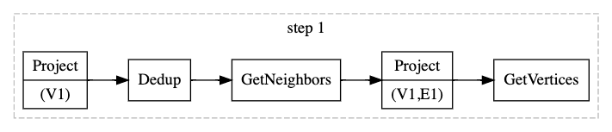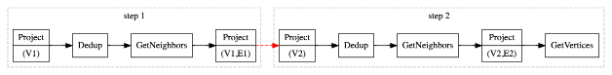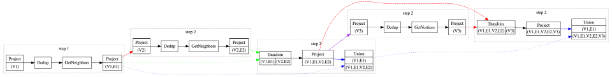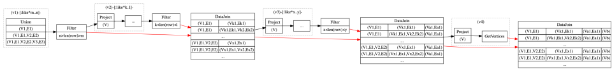source-code

# NebulaGraph Source Code Explained: Variable-Length Pattern MatchingAt the very heart of openCypher, the `MATCH` clause allows you to specify simple query patterns to retrieve the relationships from a graph database. A variable-length pattern is commonly used to describe paths and it is NebulaGraph's first try to get nGQL compatible with openCypher in the `MATCH` clause.

As can be seen from the previous articles of this series, an execution plan is composed of physical operators. Each operator is responsible for executing unique computational logics. To implement the `MATCH` clause, the operators such as `GetNeighbors`, `GetVertices`, `Join`, `Project`, `Filter`, and `Loop`, which have been introduced in the previous articles, are needed. Unlike the tree structure in a relational database, the execution process expressed by an execution plan in NebulaGraph is a cyclic graph. How to transform a variable-length pattern into a physical plan in NebulaGraph is the focus of the Planner. In this article, we will introduce how variable-length pattern matching is implemented in NebulaGraph.

## Problem Analysis

### Fixed-Length Pattern

In a `MATCH` clause, a fixed-length pattern is commonly used to search for a relationship. If a fixed-length pattern is considered a special case of the variable-length pattern, that is, a pattern describing a path of a specified length, the implementations of both can be unified. Here are the examples.

``````// Fixed-length pattern MATCH (v)-[e]-(v2)
// Variable-length pattern MATCH (v)-[e*1..1]-(v2)
``````

The preceding examples differ from each other in the type of the `e` variable. In the fixed-length pattern, `e` represents an edge, while in the variable-length one, `e` represents a list of edges of length 1.

### Connecting Variable-Length Patterns

According to the syntax of openCypher, a `MATCH` clause allows you to specify a combination of various patterns for describing complicated paths. For example, two variable-length patterns can be connected as follows.

``````MATCH (v)-[e*1..3]-(v2)-[ee*2..4]-(v3)
``````

The pattern combination in the preceding example is extendable, which means by connecting variable-length and fixed-length patterns in different ways, various complicated paths can be queried. Therefore, we must find a pattern to generate an execution plan to iterate the whole process recursively. The following conditions must be considered:

1. The following variable-length path depends on the preceding one.
2. The variables in the following pattern depend on the preceding pattern.
3. Before the next traversal step, the starting vertex must be de-duplicated.

From the following example, you can see that as long as an execution plan can be generated for the part of `()-[:like*m..n]-`, combinations and iterations may be applied to generate plans for the subsequent parts.

``````()-[:like*m..n]- ()-[:like*k..l]- ()
\____________/   \____________/   \_/
Pattern1         Pattern2       Pattern3
``````

## Execution Plan

In this section, we will introduce how the ()-[:like*m..n]- part in the preceding example is transformed into a physical execution plan in NebulaGraph. This pattern describes a graph of a minimum of `m` hops and a maximum of `n` hops. In NebulaGraph, a one-step traversal is completed by the `GetNeighbors` operator. To implement a multi-step traversal, each traversal step must call the `GetNeighbors` operator again on the basis of the previous step, and when the traversal of all the steps are completed, all the retrieved vertices and edges are connected end to end to form a single path. What users need is the paths of `m` to `n` relationships. However, in the execution process, paths of length 1 to length `n` are queried and are stored for output or for the next traversal, but only the paths of length `m` to `n` are retrieved.

### One-Step Traversal

Let's see what the one-step traversal looks like. In NebulaGraph, the source vertex is stored together with its outgoing edges, so retrieving them does not need to access data across partitions. However, the destination vertex and its incoming edges are stored in different partitions, so `GetVertices` is necessary for retrieving the properties of the vertex. In addition, to avoid replicated scanning of Storage, the source vertices must be de-duplicated before the traversal. The execution plan of a one-step traversal is shown as follows.### Multi-Step Traversal

The process of a multi-step traversal is the repetition of one-step traversal. However, we can see that the `GetNeighbors` operator can retrieve the properties of an edge's source vertex, so the `GetVertices` operator can be omitted in the previous step. Here is an execution plan of a two-step traversal.### Storing Paths

The paths retrieved in each traversal step may be needed at the end of the traversal, so all the paths must be stored. The paths for a two-step traversal are connected by the `Join` operator. In the result of the example `()-[e:like*m..n]-`, `e` represents a list of data (edges), so `Union` is needed to merge the results of each traversal step. The execution plan will be evolved further as follows.### Connecting Variable-Length Patterns

After the implementations of the preceding process, a physical plan will be generated for the `()-[e:like*m..n]-` pattern. If multiple similar patterns are connected together, such a process is iterated. However, before the iteration, the results of the previous process must be filtered to get the paths of length `m` to length `n`. The retrieved dataset of the previous process involves the paths of length 1 to length `n`, so filtering them by path length is needed. When the variable-length patterns are connected together, the execution plan becomes as follows.After the step-by-step decomposition of the patterns, the expected execution plan for the `MATCH` clause is finally generated. As you can see, it takes a lot of effort to transform a complicated pattern into the underlying interfaces for a traversal. Of course, the execution plan can be optimized, such as the multi-step traversal can be encapsulated by using the Loop operator and the sub-plan of a one-step traversal can be reused, which will not be detailed in this article. If you are interested, please refer to the source code of NebulaGraph.

## Summary

This article demonstrated the process of generating an execution plan for a `MATCH` clause with a variable-length pattern. While reading the article, you may have this question: Why such a basic and simple path query will generate such a complicated execution plan in NebulaGraph? It's not like Neo4j, where only a few operators are needed to complete the same job. In NebulaGraph, complicated directed acyclic graphs (DAG) are generated.

The answer is that in NebulaGraph, the operators are closer to the underlying interfaces and there is a lack of semantic abstractions for higher-level graph operations. The operator granularity is too fine, so too many details need to be considered to implement the optimization of the upper layer. We will further study the execution operators to gradually improve the functionality and the performance of the `MATCH` clause.

If you encounter any problems in the process of using NebulaGraph, please refer to NebulaGraph Database Manual to troubleshoot the problem. It records in detail the knowledge points and specific usage of the graph database and the graph database NebulaGraph.

Join our Slack channel if you want to discuss with the rest of the NebulaGraph community!